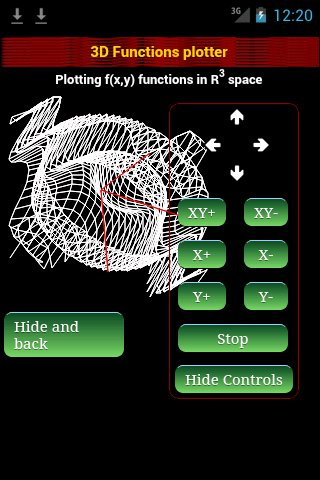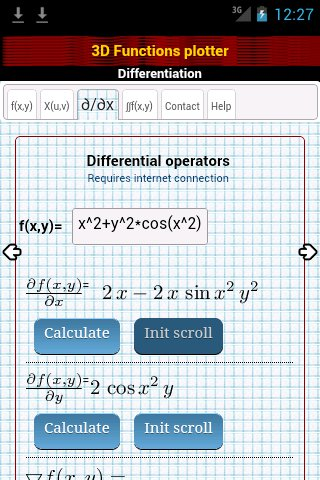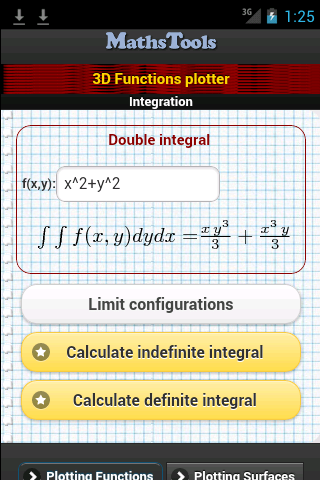# 3D-Functions Plotter Mobile for Android devices, Free

### Basics Concepts and Principles

Reject imitations! This App is free in the google market, www.mathstools.com has this unique app for multivariable analysis, it has no advertising and no charge any added values. Be careful!

3D-Functions Plotter Android version is a powerful applicacion to plot real variabled functions with two variables for Android Devices, ie functions of kind

f: R2 → R
(x,y) → f(x,y)

Also, 3D-Functions Plotter Android version calculates indefinite integrals, definite integrals, partial derivates, Laplacian and Gradient in those functions.3D-Functions Plotter makes graphics of functions f(x,y), as in this case f(x,y)=sin(x2+y2) 3D-Functions Plotter makes graphics of Regular surfaces, as in this case the Torus.3D-Functions Plotter calculates partial derivatives and several differential operators (Laplacian, Gradient ...) Example of double integration of f(x,y)=x2+y2

## Was useful? want add anything?

Post here

### A Kumar:

2012-11-07 15:24:35
Awesome app.

I can plot functions in R3 space and calculate integrals, for only 1.99\$

Very better than much others more expensives!!!

Post here
Update cookies preferences